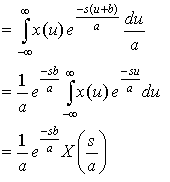## Laplace Transform Properties

As we saw from the Fourier Transform, there are a number of properties that can simplify taking Laplace Transforms. I'll cover a few properties here and you can read about the rest in the textbook.

### Real Time Shifting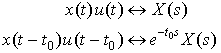Derive this:

Plugging in the time-shifted version of the function into the Laplace Transform definition, we get: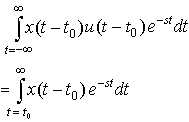Letting τ = t - t0, we get: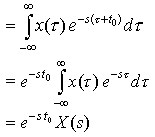Example 1  Find the Laplace Transform of x(t) = sin[b(t - 2)]u(t - 2)

### Differentiation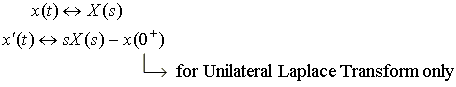Recall the equation for the voltage of an inductor: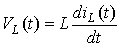If we take the Laplace Transform of both sides of this equation, we get: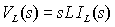which is consistent with the fact that an inductor has impedance sL.

Proof of the Differentiation Property:

1) First write x(t) using the Inverse Laplace Transform formula: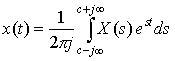2) Then take the derivative of both sides of the equation with respect to t (this brings down a factor of s in the second term due to the exponential):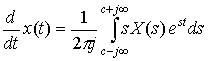3) This shows that x'(t) is the Inverse Laplace Transform of s X(s):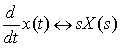The Differentiation Property is useful for solving differential equations.

### Integration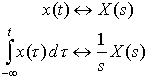Recall the equation for the voltage of a capacitor turned on at time 0: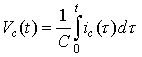If we take the Laplace Transform of both sides of this equation, we get: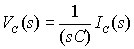which is consistent with the fact that a capacitor has impedance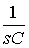.

### Multiplication by t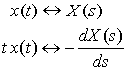Derive this: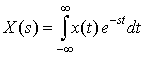Take the derivative of both sides of this equation with respect to s: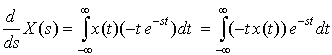This is the expression for the Laplace Transform of -t x(t). Therefore,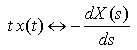### Initial Value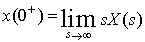(Given without proof)

### Final Value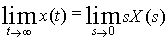(Given without proof)

### Independent-Variable Transformation (for Unilateral Laplace Transform)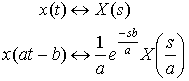Derive this:

Plugging in the definition, we find the Laplace Transform of x(at -b):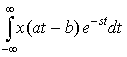Let u = at - b and du = adt, we get: